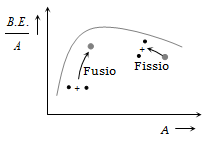Fission of nuclei is possible because the binding energy per nucleon in them
(a) Increases with mass number at high mass numbers
(b) Decreases with mass number at high mass numbers
(c) Increases with mass number at low mass numbers
(d) Decreases with mass number at low mass numbers

(b)Difficulty Level:

• 0%
• 75%
• 20%
• 5%